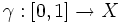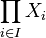# Path-connected space

(diff) ← Older revision | Latest revision (diff) | Newer revision → (diff)
This article defines a homotopy-invariant property of topological spaces, i.e. a property of homotopy classes of topological spaces

View other homotopy-invariant properties of topological spaces OR view all properties of topological spaces
This is a variation of connectedness. View other variations of connectedness

View a complete list of basic definitions in topology

## Definition

### Symbol-free definition

A topological space is said to be path-connected or arc-wise connected if given any two points on the topological space, there is a path (or an arc) starting at one point and ending at the other.

### Definition with symbols

A topological space$X$ is said to be path-connected or arc-wise connected if for any two points$a,b \in X$ there is a continuous map$\gamma:[0,1] \to X$ such that$\gamma(0) = a$ and$\gamma(1) = b$.

## Metaproperties

Metaproperty name Satisfied? Proof Statement with symbols
subspace-hereditary property of topological spaces No path-connectedness is not hereditary It is possible to have a path-connected space$X$ and a subset$A$ of$X$ such that$A$ is not path-connected in the subspace topology.
weakly hereditary property of topological spaces No path-connectedness is not weakly hereditary It is possible to have a path-connected space$X$ and a closed subset$A$ of$X$ such that$A$ is not path-connected in the subspace topology.
product-closed property of topological spaces Yes path-connectedness is product-closed Suppose$X_i, i \in I$, are all path-connected spaces. Then, the Cartesian product$\prod_{i \in I} X_i$ is also a path-connected space with the product topology.
box product-closed property of topological spaces No path-connectedness is not box product-closed It is possible to have$X_i, i \in I$ all path-connected spaces such that the Cartesian product$\prod_{i \in I} X_i$ is not path-connected in the box topology.
coarsening-preserved property of topological spaces Yes path-connectedness is coarsening-preserved If$X$ is path-connected under a topology$\tau$, it remains path-connected when we pass to a coarser topology than$\tau$.
continuous image-closed property of topological spaces Yes path-connectedness is continuous image-closed If$X$ is a path-connected space and$Y$ is the image of$X$ under a continuous map, then$Y$ is also path-connected.
connected union-closed property of topological spaces Yes path-connectedness is connected union-closed
closure-preserved property of topological spaces No path-connectedness is not closure-preserved It is possible to have a$A$ a subset of$X$ that is path-connected in the subspace topology but such that the closure$\overline{A}$ is not path-connected in its subspace topology.